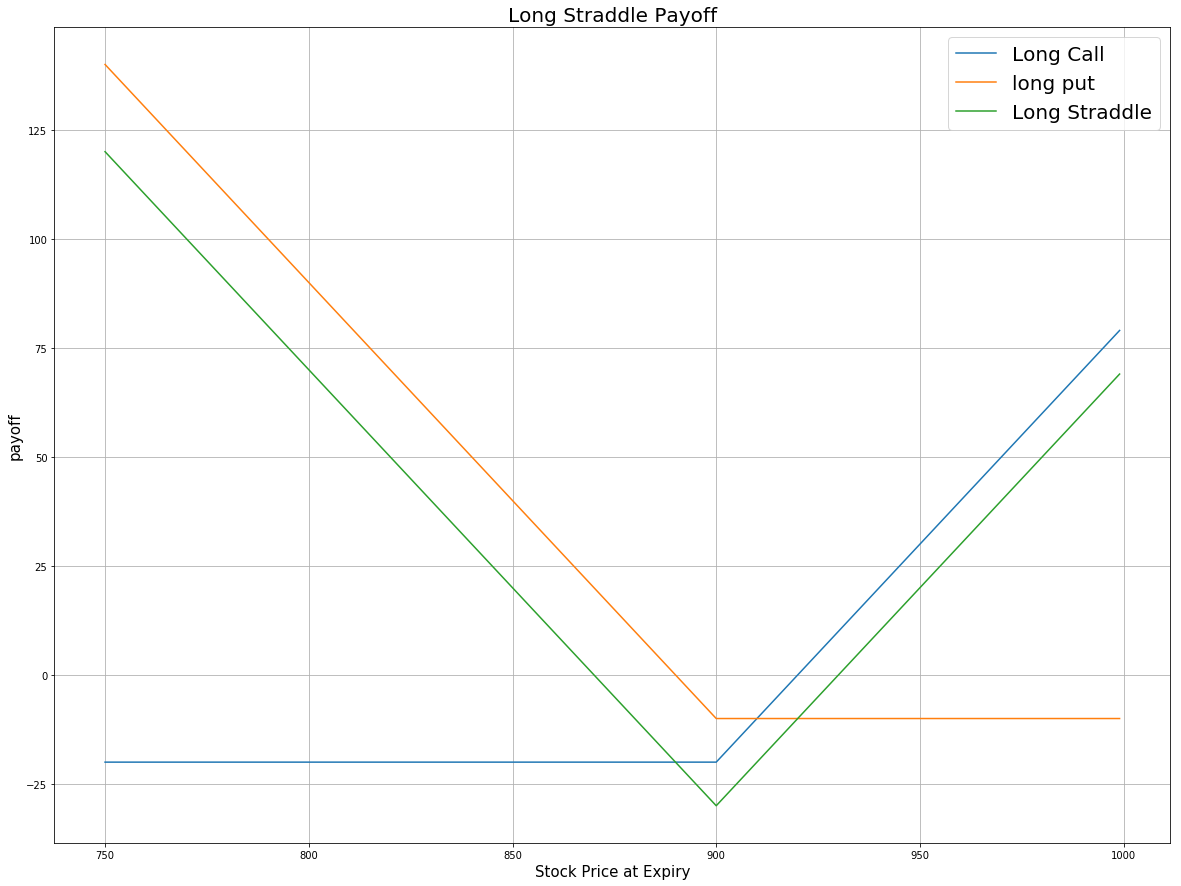# Option Strategies

### Introduction

Long Straddle is an Options trading strategy that consists of buying an ATM call and an ATM put, where both contracts have the same underlying asset, strike price, and expiration date. This strategy aims to profit from volatile movements in the underlying stock, either positive or negative.

### Implementation

1. In the Initialize method, set the start date, end date, cash, and Option universe.
2. private Symbol _symbol;

public override void Initialize()
{
SetStartDate(2017, 4, 1);
SetEndDate(2017, 6, 30);
SetCash(100000);

_symbol = option.Symbol;
option.SetFilter(-1, 1, TimeSpan.FromDays(30), TimeSpan.FromDays(60));
}
def Initialize(self) -> None:
self.SetStartDate(2017, 4, 1)
self.SetEndDate(2017, 6, 30)
self.SetCash(100000)

self.symbol = option.Symbol
option.SetFilter(-1, 1, timedelta(30), timedelta(60))
3. In the OnData method, select the expiration date and strike price of the contracts in the strategy legs.
4. public override void OnData(Slice slice)
{
if (Portfolio.Invested) return;

// Get the OptionChain
var chain = slice.OptionChains.get(_symbol, null);
if (chain == null || chain.Count() == 0) return;

// Select an expiration date
var expiry = chain.OrderBy(contract => contract.Expiry).Last().Expiry;

// Select the ATM strike price
var strike = chain.Where(contract => contract.Expiry == expiry)
.Select(contract => contract.Strike)
.OrderBy(strike => Math.Abs(strike - chain.Underlying.Price))
.First();
def OnData(self, slice: Slice) -> None:
if self.Portfolio.Invested: return

# Get the OptionChain
chain = slice.OptionChains.get(self.symbol, None)
if not chain: return

# Select an expiration date
expiry = sorted(chain, key=lambda contract: contract.Expiry, reverse=True).Expiry

# Select the ATM strike price
strikes = [contract.Strike for contract in chain if contract.Expiry == expiry]
strike = sorted(strikes, key=lambda strike: abs(strike - chain.Underlying.Price))
5. In the OnData method, call the OptionStrategies.Straddle method and then submit the order.
6. var optionStrategy = OptionStrategies.Straddle(_symbol, strike, expiry);
Buy(optionStrategy, 1);
option_strategy = OptionStrategies.Straddle(self.symbol, strike, expiry)
self.Buy(option_strategy, 1)

Option strategies synchronously execute by default. To asynchronously execute Option strategies, set the asynchronous argument to Falsefalse. You can also provide a tag and order properties to the Buy methods.

Buy(optionStrategy, quantity, asynchronous, tag, orderProperties);

self.Buy(option_strategy, quantity, asynchronous, tag, order_properties)


### Strategy Payoff

The payoff of the strategy is

$$\begin{array}{rcll} C^{ATM}_T & = & (S_T - K^{C})^{+}\\ P^{ATM}_T & = & (K^{P} - S_T)^{+}\\ P_T & = & (C^{ATM}_T + P^{ATM}_T - C^{ATM}_0 - P^{ATM}_0)\times m - fee \end{array}$$ $$\begin{array}{rcll} \textrm{where} & C^{ATM}_T & = & \textrm{ATM call value at time T}\\ & P^{ATM}_T & = & \textrm{ATM put value at time T}\\ & S_T & = & \textrm{Underlying asset price at time T}\\ & K^{C} & = & \textrm{ATM call strike price}\\ & K^{P} & = & \textrm{ATM put strike price}\\ & P_T & = & \textrm{Payout total at time T}\\ & C^{ATM}_0 & = & \textrm{ATM call value at position opening (debit paid)}\\ & P^{ATM}_0 & = & \textrm{ATM put value at position opening (debit paid)}\\ & m & = & \textrm{Contract multiplier}\\ & T & = & \textrm{Time of expiration} \end{array}$$

The following chart shows the payoff at expiration:The maximum profit is unlimited if the underlying price rises to infinity at expiration.

The maximum loss is the net debit paid, $C^{ATM}_0 + P^{ATM}_0$. It occurs when the underlying price is the same at expiration as it was when you opened the trade. In this case, both Options expire worthless.

### Example

The following table shows the price details of the assets in the algorithm at Option expiration (2017-05-20):

AssetPrice ($)Strike ($)
Call22.30835.00
Put23.90835.00
Underlying Equity at expiration934.01-

Therefore, the payoff is

$$\begin{array}{rcll} C^{ATM}_T & = & (S_T - K^{C})^{+}\\ & = & (934.01-835.00)^{+}\\ & = & 98.99\\ P^{ATM}_T & = & (K^{P} - S_T)^{+}\\ & = & (835.00-934.01)^{+}\\ & = & 0\\ P_T & = & (C^{ATM}_T + P^{ATM}_T - C^{ATM}_0 - P^{ATM}_0)\times m - fee\\ & = & (98.99+0-22.3-23.9)\times100-1.00\times2\\ & = & 5277 \end{array}$$

So, the strategy gains \$5,277.

The following algorithm implements a long straddle Option strategy:

You can also see our Videos. You can also get in touch with us via Discord.